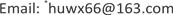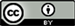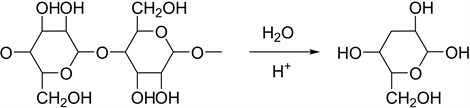﻿ 分子文物学 Molecular Cultural Relicology

Interdisciplinary Science Letters
Vol. 03  No. 01 ( 2019 ), Article ID: 28944 , 5 pages
10.12677/ISL.2019.31002

Molecular Cultural Relicology

Fangfang Zou1,2, Xingcheng Zhang3, Luyao Wang4, Wenxiang Hu1,2,5*

1School of Chemical Engineering and Pharmacy, Wuhan Institute of Technology, Wuhan Hubei

2Jingdong Xianghu Microwave Chemistry Union Laboratory, Beijing Excalibur Space Military Academy of Medical Sciences, Beijing

3College of Chemistry and Chemical Engineering, Xinyang Normal University, Xinyang Henan

4Department of Chemistry, Capital Normal University, Beijing

5Space Systems Division, Strategic Support Troops, Chinese People’s Liberation Army, BeijingReceived: Jan. 31st, 2019; accepted: Feb. 14th, 2019; published: Feb. 21st, 2019ABSTRACT

The preservation environment of cultural relics is one of the main factors of cultural relic damage. This paper expounds various factors of the damage to cultural relics, such as temperature, humidity, air pollutants and illumination, discusses the corrosion mechanism of bronzes, ancient paintings and stone artifacts in detail, and summarizes the methods of cultural relic protection.

Keywords:Cultural Relic, Corrosion Molecular Mechanism, Protection

1武汉工程大学化工与制药学院，湖北 武汉

2北京神剑天军医学科学院京东祥鹄微波化学联合实验室，北京

3信阳师范学院化学与化学工程学院，河南 信阳

4首都师范大学化学系，北京

5中国人民解放军战略支援部队航天系统部，北京1. 引言

2. 影响文物腐蚀的因素

2.1. 温度和湿度

2.2. 空气污染物

2.3. 光照

3. 文物腐蚀分子机理

3.1. 青铜器的腐蚀

$\text{Cu}+{\text{O}}_{\text{2}}+{\text{H}}_{\text{2}}\text{O}+{\text{CO}}_{\text{2}}\to \text{Cu}{\left(\text{OH}\right)}_{\text{2}}\cdot \text{CuCO}$

$\text{Cu}+{\text{Cl}}^{-}\to \text{CuCl}+\text{e}$

$\begin{array}{l}\text{Cu}+{\text{Cl}}^{-}\to \text{CuCl}+\text{e}\\ \text{2CuCl}+{\text{H}}_{\text{2}}\text{O}\to {\text{Cu}}_{\text{2}}\text{O}+\text{2HCl}\\ {\text{Cu}}_{\text{2}}\text{O}+\text{1}/{\text{2O}}_{\text{2}}\text{+}{\text{H}}_{\text{2}}\text{O}+{\text{CO}}_{\text{2}}\to {\text{CuCO}}_{\text{3}}\cdot \text{Cu}{\left(\text{OH}\right)}_{\text{2}}\\ {\text{Cu}}_{\text{2}}\text{O}+2{\text{H}}_{\text{2}}\text{O}+{\text{O}}_{\text{2}}+2\text{HCl}\to {\text{CuCl}}_{2}\cdot 3\text{Cu}{\left(\text{OH}\right)}_{\text{2}}\\ \text{4CuCl}+{\text{O}}_{\text{2}}+4{\text{H}}_{2}\text{O}\to {\text{CuCl}}_{2}\cdot 3\text{Cu}{\left(\text{OH}\right)}_{\text{2}}+2\text{HCl}\end{array}$

3.2. 古书画的损毁3.3. 石质文物

$\text{2K}\left[{\text{AlSi}}_{\text{3}}{\text{O}}_{\text{6}}\right]+{\text{CO}}_{\text{2}}+{\text{2H}}_{\text{2}}\text{O}={\text{Al}}_{\text{2}}{\text{Si}}_{\text{2}}{\text{O}}_{\text{5}}{\left(\text{OH}\right)}_{\text{4}}+{\text{K}}_{\text{2}}{\text{CO}}_{\text{3}}+{\text{SiO}}_{\text{2}}$

4. 文物的保护与修复

5. 结束语

Molecular Cultural Relicology[J]. 交叉科学快报, 2019, 03(01): 6-10. https://doi.org/10.12677/ISL.2019.31002

1. 1. 田金英, 王方, 隗亚林. 环境对文物的影响及故宫博物院的环境调查[J]. 中国博物馆, 1994(1): 76-82.

2. 2. 杨利广. 博物馆纸质文物的保存现状及病害原因分析[J]. 丝绸之路, 2016(24): 65-66.

3. 3. 毕建洪. 青铜文物腐蚀与保护研究[J]. 安徽大学学报(自科版), 2007, 31(4): 77-80.

4. 4. 刘舜强. 古书画损毁机理初探[J]. 文物保护与考古科学, 2003, 15(1): 39-42.

5. 5. 张金风. 石质文物病害机理研究[J]. 文物保护与考古科学, 2008, 20(2): 60-67.

6. 6. 王蕙贞, 冯楠, 宋迪生. 高句丽石质文物风化的保护方法研究[J]. 文博, 2010(6): 76-81.

7. 7. 徐方圆,邱建辉,孙振乾.含氟聚合物加固保护纸质文物研究[J]. 文物保护与考古科学, 2004, 16(4):1-5.

8. 8. Vohrer, U., Trick, I., Bern-hardt, J., et al. (2001) Plasma Treatment—An Increasing Technology for Paper Restoration? Surface & Coatings Technology, 142, 1069-1073. https://doi.org/10.1016/S0257-8972(01)01280-4

9. 9. Huang, Z.W. (2011) Applica-tion of Chemicals in the Restoration of Monuments. Advanced Materials Research, 374-377, 1442-1445. https://doi.org/10.4028/www.scientific.net/AMR.374-377.1442

10. 10. 科学家用细菌保护珍贵古画免受以色素为食微生物破坏[EB/OL]. https://baijiahao.baidu.com/s?id=1619175731648052877&wfr=spider&for=pc

11. 11. 秦宁, 闵清, 胡文祥. 分子雨水学[J]. 交叉科学快报, 2018, 2(3): 88-91. https://doi.org/10.12677/ISL.2018.23017

12. NOTES

*通讯作者。